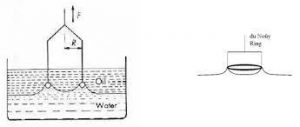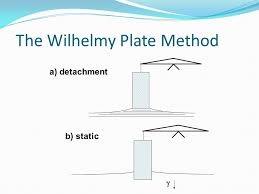# Measurement of Surface and Interfacial tension, Ring Detachment Method (du Nouy tensiometer) and Wilhelmy Plate Method and MCQs for GPAT, NIPER, Pharmacist and Drug Inspector exam

## Measurement of Surface and Interfacial tension, Ring Detachment Method (du Nouy tensiometer) and Wilhelmy Plate Method and MCQs for GPAT, NIPER, Pharmacist and Drug Inspector exam

Ring Detachment Method (du Nouy tensiometer):

The du Nouy’s ring method is similar to the Wilhelmy plate method, but it uses a ring or loop of wire. A platinum or Iridium wire of ~4 cm in circumference is suspended from the loop attached to a scale through a torsion wire. The liquid, whose surface tension is to be determined, is taken in a container. The position of the container is adjusted so that the ring just touches the surface of the liquid. The torsion on the wire is increased gradually so that the ring just detaches from the surface of the liquid.Fig 1 – Du Nuoy tensiometer (taken from standard test method for interfacial tension mastrad test systems)

Again, the detachment force is equal to the surface tension multiplied by the perimeter of liquid detached; hence:

F = W + 4πry

Harkins and Jordan introduced a correction factor f (function of meniscus volume V and radius r of the wire) for more accurate calculation of ᵞ.

F = ᵞ / ᵞideal = (R3/V, R/r)

A correction factor is required before accurate results can be obtained since the above equation does not take into account variables such as the radius of the ring (R), the radius of the wire used to form the ring (r) and the volume of the liquid supported by the ring during detachment (V).

1. The ring should lie horizontally in the surface and the wire used to prepare the ring should be absolutely clean.
2. The vessel containing the liquid should be large to minimize the influence of the shape of the liquid raised by the ring.
3. The surface of the liquid should be free from wave motion.
4. Temperature control should be adequate.

Wilhelmy Plate Method:

Principle: The force necessary to detach a plate from the surface or interface of two immiscible liquids is proportional to the surface or interfacial tension, respectively.

The apparatus consists of a thin plate made from glass, mica or platinum attached to a suitable balance. The plate is either detached from the interface (nonequilibrium condition) or its weight is measured statically using an accurate microbalance.

In the detachment method, the plate is immersed in the liquid (whose surface tension is to be measured) taken in a container. The liquid container is then gradually lowered and the reading on the balance immediately prior to detachment is noted. The total force (reading on the balance) F is given by the weight of the plate W and the interfacial tension force.

F = W + ᵞp

where p is the ‘contact length’ of the plate with the liquid, i.e. the plate perimeter. Thus, the detachment force is equal to the surface tension multiplied by the perimeter of the surface detached:Fig 2 – Wilhelmy plate method (taken from colloid systems myshare.ru)

F – W = 2(L + T)ᵞ

Or

ᵞ = F – W / 2(L + T)

where L and T are the length and thickness of the plate, respectively.

In the static technique, the plate is suspended from one arm of a microbalance and the whole vessel containing the two liquid layers is raised until the interface touches the plate or alternatively the plate is allowed to penetrate the upper liquid layer until it touches the interface. The increase in weight ‘W is given by the following equation:

∆W = ᵞpcosⱷ

Where ⱷ is the contact angle. If the plate is completely wetted by the lower liquid as it penetrates, ⱷ is 0 and  ᵞ may be calculated directly from ∆W. The static technique is useful for assessing changes in interfacial tension as a function of time.

1. A roughened platinum or glass plate is used to ensure a zero contact angle.
2. If the oil is denser than water, a hydrophobic plate is used to ensure its complete wetting.

Multiple choice questions (MCQs)

1.Ring Detachment Method is also known as

a)du Nouy tensiometer

b)Wilhelmy plate method

c)Drop count method

d)Drop weight method

2.Gegenions means

a)Amphiphiles

b)Ions having a charge opposite to the potential determining ions

c)Ions having same charge as that of potential determining ions

d)Potential determining ions

3.A ring or loop of wire used in Ring Detachment Method is made up of

a)Platinum

b)Iridium

c)Both of these

d)None of these

4.What is the circumference of wire used in Ring Detachment Method?

a)2 cm

b)3 cm

c)4 cm

d)5 cm

5.Who introduced a correction factor f (function of meniscus volume V and radius r of the wire) for more accurate calculation of ᵞ?

a)Harkins

b)Jordan

c)Both of these

d)None of these

6.The force necessary to detach a plate from the surface or interface of two immiscible liquids is proportional to the surface or interfacial tension, respectively. This principle is involved in

a)Wilhelmy plate method

b)Drop count method

c)Du Nuoy tensiometer

d)All of the above

7.The apparatus used in Wilhelmy plate method consists of a thin plate made from

a)Glass

b)Mica

c)Platinum

d)All of the above

8.Which of the following are types of Wilhelmy plate method?

a)Detachment method

b)Static method

c)Both of these

d)None of these

9.Which of the following equation is used in Wilhelmy plate method?

a)ᵞ = F – W / 2(L + T)

b)F = W + 4πry

c)∆W = ᵞpcosⱷ

d)F = ᵞ / ᵞideal = (R3/V, R/r)

10.In Ring Detachment Method the contact angle is controlled by

a)Complete wetting

b)Partial wetting

c)Incomplete wetting

d)No wetting

11.Surface tension of water at 25ºC is

a)58dynes/cm

b)68dynes/cm

c)72dynes/cm

d)82dynes/cm

12.Interfacial tension will not exist between

a)Solid-solid

b)Solid-liquid

c)Liquid-liquid

d)Gas-gas

13.ᵞ = F – W / 2(L + T). In this equation L is

a)length of the plate

b)thickness of the plate

c)height of the plate

d)width of the plate

14.ᵞ = F – W / 2(L + T). In this equation T is

a)length of the plate

b)thickness of the plate

c)height of the plate

d)width of the plate

15.In ∆W = ᵞpcosⱷ, ⱷ is

a)contact angle

b)angle of repose

c)both of these

d)none of these

Solutions:

1. a)du Nouy tensiometer
2. b) Ions having a charge opposite to the potential determining ions
3. c)Both of these
4. c)4 cm
5. c)Both of these
6. a)Wilhelmy plate method
7. d)All of the above
8. c)Both of these
9. a)ᵞ = F – W / 2(L + T)
10. a)Complete wetting
11. c) 72dynes/cm
12. d) Gas-gas
13. a)length of the plate
14. b)thickness of the plate
15. a)contact angle

References:

1. GAURAV KUMAR JAIN – THEORY & PRACTICE OF PHYSICAL PHARMACY, 1st edition 2012 Elsevier, page no. 116-118.

2. martins Physical Pharmacy, 6th edition 2011, page no. 662-664.

For More Standard and Quality Question Bank you can Join Our Test Series Programme for GPAT, NIPER JEE, Pharmacist Recruitment Exam, Drug Inspector Recruitment Exams, PhD Entrance Exam for Pharmacy

List of Successful GPATINDIAN CANDIDATES